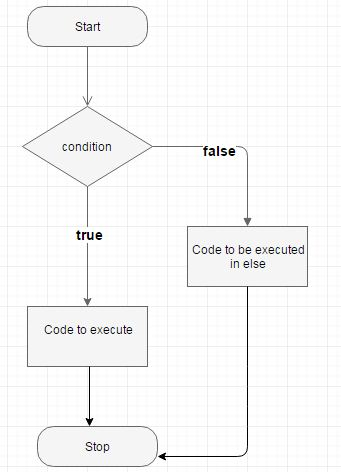### Java If-else Statement Example Program

Java if-else statement is used to execute some code when condition is true,when condition is false other code  will execute which is in else statement.

#### If-else structure

```if(condition){
//executes if condition is true
}else{
//executes if condition is false
}```#### Example

```public class IfExample{
private int x;

public void ifCheck(int x) {
if (x % 2 == 0) {
System.out.println("The number is even ");
}else{
System.out.println("The number is odd ");
}
}

public static void main(String[] args) {
IfExample i = new IfExample();
i.ifCheck(10);

}
}```